# JP6002557B2 - Optical multilevel signal pre-equalization circuit, optical multilevel signal pre-equalization transmitter, and polarization multiplexed optical pre-equalization transmitter - Google Patents

Optical multilevel signal pre-equalization circuit, optical multilevel signal pre-equalization transmitter, and polarization multiplexed optical pre-equalization transmitter Download PDF

## Info

Publication number
JP6002557B2
JP6002557B2 JP2012259883A JP2012259883A JP6002557B2 JP 6002557 B2 JP6002557 B2 JP 6002557B2 JP 2012259883 A JP2012259883 A JP 2012259883A JP 2012259883 A JP2012259883 A JP 2012259883A JP 6002557 B2 JP6002557 B2 JP 6002557B2
Authority
JP
Japan
Prior art keywords
pre
circuit
optical
signal
equalization
Prior art date
Legal status (The legal status is an assumption and is not a legal conclusion. Google has not performed a legal analysis and makes no representation as to the accuracy of the status listed.)
Active
Application number
JP2012259883A
Other languages
Japanese (ja)
Other versions
JP2014107736A (en
Inventor

Original Assignee

Priority date (The priority date is an assumption and is not a legal conclusion. Google has not performed a legal analysis and makes no representation as to the accuracy of the date listed.)
Filing date
Publication date
Application filed by 株式会社日立製作所 filed Critical 株式会社日立製作所
Priority to JP2012259883A priority Critical patent/JP6002557B2/en
Publication of JP2014107736A publication Critical patent/JP2014107736A/en
Application granted granted Critical
Publication of JP6002557B2 publication Critical patent/JP6002557B2/en
Application status is Active legal-status Critical
Anticipated expiration legal-status Critical

## Images

•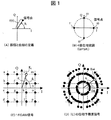•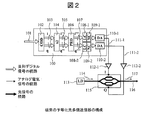•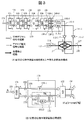•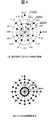••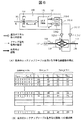•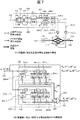•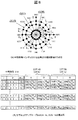•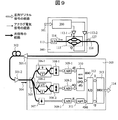••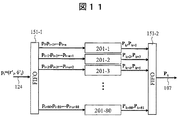•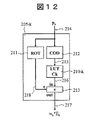•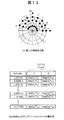•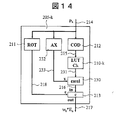••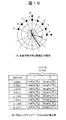••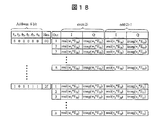•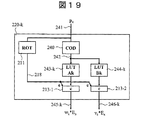••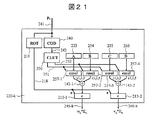•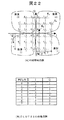••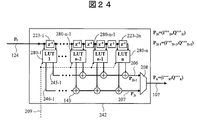## Classifications

• HELECTRICITY
• H04ELECTRIC COMMUNICATION TECHNIQUE
• H04BTRANSMISSION
• H04B10/00Transmission systems employing electromagnetic waves other than radio-waves, e.g. infrared, visible or ultraviolet light, or employing corpuscular radiation, e.g. quantum communication
• H04B10/25Arrangements specific to fibre transmission
• H04B10/2507Arrangements specific to fibre transmission for the reduction or elimination of distortion or dispersion
• H04B10/2513Arrangements specific to fibre transmission for the reduction or elimination of distortion or dispersion due to chromatic dispersion
• HELECTRICITY
• H04ELECTRIC COMMUNICATION TECHNIQUE
• H04BTRANSMISSION
• H04B10/00Transmission systems employing electromagnetic waves other than radio-waves, e.g. infrared, visible or ultraviolet light, or employing corpuscular radiation, e.g. quantum communication
• H04B10/25Arrangements specific to fibre transmission
• H04B10/2507Arrangements specific to fibre transmission for the reduction or elimination of distortion or dispersion
• HELECTRICITY
• H04ELECTRIC COMMUNICATION TECHNIQUE
• H04BTRANSMISSION
• H04B10/00Transmission systems employing electromagnetic waves other than radio-waves, e.g. infrared, visible or ultraviolet light, or employing corpuscular radiation, e.g. quantum communication
• H04B10/50Transmitters
• H04B10/516Details of coding or modulation
• H04B10/54Intensity modulation
• H04B10/541Digital intensity or amplitude modulation
• HELECTRICITY
• H04ELECTRIC COMMUNICATION TECHNIQUE
• H04BTRANSMISSION
• H04B2210/00Indexing scheme relating to optical transmission systems
• H04B2210/25Distortion or dispersion compensation
• H04B2210/254Distortion or dispersion compensation before the transmission line, i.e. pre-compensation

## Description

The present invention relates to an optical information transmission technique, for example, an optical multilevel signal pre-equalization circuit, an optical multilevel signal pre-equalization transmitter, and a polarization multiplexed optical pre-equalization transmission suitable for transmission / reception of an optical multi-level signal through an optical fiber. Related to the vessel.

The amount of information (transmission capacity) that can be transmitted with a single optical fiber has almost reached the limit by almost using the wavelength band of the optical fiber amplifier due to the increase in the number of wavelengths and the increase in the modulation speed of the optical signal. Furthermore, in order to increase the transmission capacity of an optical fiber, it is necessary to devise a signal modulation method, pack a large number of optical signals in a limited frequency band, and increase the use efficiency of the frequency band. The use of is being considered. Many studies have been reported so far. For example, Non-Patent Document 1 reports QPSK (Quadrature Phase Shift Keying) that performs quaternary phase modulation. Non-Patent Document 2 reports long-distance transmission of 16QAM signals using optical delay detection, which is a type of direct detection. Non-Patent Document 3 reports a 16QAM signal coherent reception method using polarization multiplexing. Non-Patent Document 4 reports a method of receiving and coherently receiving a 16-level optical multilevel signal and then digitally performing delay detection in the receiver.

FIG. 1 shows a complex phase plane (or complex plane, phase plane, IQ plane) used for optical transmission, and signal point arrangements of various known modulation schemes. In FIG. 1, signal points of various optical multilevel signals (complex display of an optical electric field at an identification time) are plotted on the complex phase plane. FIG. 1A shows signal points on the IQ plane. Each signal point can be displayed in complex orthogonal coordinates (IQ coordinates) or polar coordinates indicated by amplitude r (n) and phase φ (n) shown in the figure. (B) of FIG. 1 uses two values (0, π / 2, π, −π / 2) as the phase angle φ (n), and 2-bit information (00, 01, 11, 10) using one symbol. ) Shows four-level phase modulation (QPSK). FIG. 1C shows 16-value quadrature amplitude modulation (16QAM) widely used in radio. In 16QAM, signal points are arranged in a lattice pattern, and 4-bit information transmission is possible with one symbol. FIG. 1D shows a signal point arrangement of a 16QAM signal that has been subjected to phase pre-integration processing, which is signal processing on the transmission side that cancels the effect of delayed detection, by the methods shown in Non-Patent Document 2 and Non-Patent Document 4 described above. Is shown.

Optical delay detection and delay detection at the electrical signal stage are processes for detecting a phase difference by interfering two temporally continuous symbols, and the phase component of the output signal from the delay detector is temporally continuous. The phase difference Δφ between the two symbols. For this reason, when a multilevel signal such as a 16QAM signal is used, the signal output from the delay detector on the receiving side has a signal point arrangement with intersymbol interference that is significantly different from the original multilevel signal. In addition, the amplitude component after delay detection is also the product of the amplitudes of two symbols that are temporally continuous.

In order to prevent such intersymbol interference from occurring, Non-Patent Document 2 introduces a phase pre-integration process. This calculation is a process of integrating the phase of the optical signal for each symbol in advance on the transmission side. The phase of each symbol n is φ (n), the signal amplitude r (n) is left as it is, and the phase of the signal is Σφ ( It is a process to replace with n).

The signal point arrangement of the 16QAM signal subjected to the phase pre-integration processing is greatly different from the original signal point arrangement as shown in FIG. 1D, but the phase pre-integration (phase addition processing) is performed by the receiver. The phase component of the signal output from the delay detector is the same as the phase component of the original 16QAM signal. Therefore, by combining this phase component and the separately detected amplitude component r (n) in the receiver, the signal point arrangement of the original 16QAM signal in FIG. 1C can be reproduced. As described above, when the phase pre-integration processing on the transmission side is used, even when using an optical receiver using optical delay detection or delay detection at an electric signal stage, it is possible to use a multilevel signal having an arbitrary signal point arrangement. it can.

On the other hand, in ultrahigh-speed optical fiber transmission, phenomena such as chromatic dispersion, polarization dispersion, and band limitation of optical fibers and components used are major limiting factors for increases in transmission speed and transmission distance. “Chromatic dispersion (CD)” is a phenomenon in which light of different wavelengths is transmitted at different speeds in an optical fiber. The optical spectrum of the optical signal modulated at high speed includes different wavelength components, and these components arrive at the receiving end at different times due to the influence of dispersion. As a result, it is known that the optical waveform after transmission causes a large waveform distortion.

In order to avoid such influence of dispersion, a technique called chromatic dispersion compensation (hereinafter simply referred to as dispersion compensation means chromatic dispersion compensation) is being studied. Chromatic dispersion compensation means that an optical device having a wavelength dispersion characteristic opposite to that of an optical fiber used in a transmission path is arranged in an optical transmitter or optical receiver, thereby canceling the wavelength dispersion characteristic of the optical fiber and reducing the optical waveform. This is a technique for preventing distortion.

Conventionally, a method using a dispersion compensating fiber, an optical interferometer, an optical circuit, an optical fiber grating, or the like having a chromatic dispersion opposite in sign to the transmission line has been studied. However, these techniques require extra cost for the optical devices described above, and in recent years, digital signal processing has not been applied due to the limitation of the amount of chromatic dispersion and the amount of variable obtained by the optical devices. It is being considered.

Optical pre-equalization (pre-distortion) transmission is a method in which this optical electric field equalization is performed by electrical signal processing on the transmission side, and an inverse function of chromatic dispersion is applied to the optical signal electric field in advance in the transmitter. In this method, a generated optical electric field waveform is generated and transmitted through an optical fiber to cancel the influence of chromatic dispersion of the optical fiber itself. The basic concept of such a pre-equalization method has existed for several tens of years ago, but recently, a digital pre-equalization optical transmitter that implements pre-equalization by high-speed digital signal processing has been proposed. The details are shown in Non-Patent Document 5, for example. Note that the pre-equalization signal processing dealt with in the present specification is somewhat broad, and includes processing such as band narrowing and interpolation, which are linear filtering required on the transmission side in optical multilevel transmission.

FIG. 2 shows a basic configuration of a conventional pre-equalized optical multilevel transmitter that pre-equalizes the influence of chromatic dispersion. A conventional optical multilevel transmitter signal processing circuit 100 inputs an input information signal 101 to a multilevel encoding circuit 102, and digitally outputs it on an orthogonal complex plane as in a 16QAM signal ((C) in FIG. 1). Is converted into a multi-value signal sequence 103 of 1 sample / symbol expressed in (1). Next, the double interpolation circuit 104 converts the multilevel signal sequence 103 into a 2-sample / symbol multilevel signal sequence 105 by oversampling processing. The multilevel signal sequence 105 is input to a pre-equalization circuit 106 that pre-equalizes the influence of chromatic dispersion. The pre-equalization circuit 106 superimposes the inverse function of the chromatic dispersion characteristic of the transmission line on the multilevel signal sequence 105 by digital pre-equalization processing. The real part and the imaginary part of the multilevel signal 107 after pre-equalization are input to the linear response compensation circuits 108-1 and 108-2, the non-linear response compensation circuits 109-1 and 109-2, and the like. The linear response compensation circuits 108-1 and 108-2 and the non-linear response compensation circuits 109-1 and 109-2 execute digital signal processing that provides inverse compensation for linear / non-linear response deterioration of subsequent driver amplifiers and optical modulators. . The real part and the imaginary part of the digital multilevel signal generated by this signal processing are input to the DA converters 110-1 and 110-2, respectively, and converted into high-speed analog modulation signals 111-1 and 111-2. . The analog modulation signals 111-1 and 111-2 are amplified to a desired amplitude by the driver amplifiers 112-1 and 112-2 and then input to the two modulation input terminals (I and Q) of the optical IQ modulator 115. The The optical IQ modulator 115 converts the unmodulated laser light 114 output from the laser light source 113 into an optical multilevel signal 117 that has been pre-equalized to chromatic dispersion, and outputs it from the output optical fiber 116.

FIG. 3 shows a conventional configuration example of a pre-equalized optical multilevel transmitter using a phase pre-integration process. The transmitter side of the present system executes a phase pre-integration process and transmits an optical multilevel signal. On the other hand, after the optical multilevel signal is subjected to optical delay detection or coherent detection, the receiver side of this system alleviates various degradations associated with optical multilevel transmission by performing delay detection by digital calculation. Details of this method are described in Non-Patent Document 2 and Non-Patent Document 4.

The phase pre-integration process is a calculation process in which the phase components (angles) of the multilevel signal are cumulatively added for each symbol. However, if the phase pre-integration process is applied as it is to a two-dimensional multilevel signal such as 16QAM, (1) the process of converting the signal point position expressed in orthogonal coordinates to polar coordinates, and (2) the extracted phase information Complicated arithmetic processing such as processing of adding with high accuracy such as 8 to 12 bits and (3) processing of converting the signal after the addition into an orthogonal coordinate in combination with amplitude again is required. These processes can be solved by an arithmetic process using an intermediate code described in Patent Document 1. Hereinafter, the phase pre-integration processing of FIG. 3 will be described according to the configuration of Patent Document 1.

FIG. 3A shows an internal configuration of the signal processing circuit 120 of the conventional phase pre-integrated light multilevel transmission unit. In the case of the signal processing circuit 120, the information signal 101 is input to an encoding circuit 121 using an intermediate polar coordinate code and converted into a polar coordinate multilevel intermediate code (amplitude N state, phase M state) 122. The polar coordinate multilevel intermediate code 122 is input to the phase pre-integration circuit 123. The phase pre-accumulation circuit 123 executes a pre-accumulation calculation that sequentially accumulates and adds the intermediate codes of the phase portion, and then combines with the intermediate code of the original amplitude portion again to obtain an intermediate code (amplitude N state, phase M) after the phase pre-integration. State) 124. The intermediate code after the phase pre-accumulation is orthogonally transformed by the orthogonal coordinate conversion circuit 125 to become a multilevel signal 126 after the phase pre-accumulation. The subsequent configuration of the transmitter is the same as that in FIG. 2, and an optical multilevel signal 127 subjected to phase pre-integration and chromatic dispersion pre-equalization is obtained from the output optical fiber 116.

The first reason for adopting the configuration using the intermediate code as described above is that the multi-value signal is encoded with polar coordinates from the beginning, thereby omitting the polar coordinate conversion necessary for the phase pre-integration processing. The second reason is that the number of states of the multilevel signal is greatly limited (the number of amplitude states N, the number of phase states M, N, and M are integers of about 4 to 32, for example), and subsequent phase pre-integration. This is because the calculation amount of the circuit 123 and the orthogonal coordinate conversion circuit 125 is reduced.

FIG. 3B shows an example of the configuration of the conventional phase pre-accumulation circuit 123. The input polar coordinate multi-level intermediate code 122 is an amplitude intermediate code 130 of state number N and a phase information signal 131 of state number M. To be separated. The amplitude intermediate code 130 is delayed by the delay circuit 133-1. The phase information signal 131 is input to the integrating circuit 132. The adding circuit 136 of the integrating circuit 132 adds the input phase information signal φ i and the phase integrated value Σφ i-1 up to one symbol before, and the result is the pre-integrated phase information signal 134 (Σφ i ). Output as.

The number of states of the phase information signal is M. For this reason, the addition circuit 136 only needs to perform addition using M as a modulus, and the circuit scale required for addition can be reduced. Subsequently, a multi-level signal having N amplitude states and M phase states is input to the orthogonal coordinate conversion circuit 125. The orthogonal coordinate conversion circuit 125 is realized using a lookup table (LUT) or the like.

FIG. 4A shows an example of the 16QAM signal disclosed in Patent Document 1 and its intermediate code. In this example, the amplitude is 3 values and the phase is 16 values. For this reason, the amplitude of the intermediate code is represented by 2 bits, and 00 is assigned to the minimum value r1, 01 is assigned to the intermediate value r2, and 10 is assigned to the maximum value r3. The number of states of the intermediate code phase is M = 16, and the phase of the intermediate code is displayed as 16 4-bit values from 0000 to 1111.

FIG. 4B shows the signal point arrangement after the phase pre-integration. In FIG. 4B, the phase state of the 16QAM signal is limited to a position that is an integer multiple of 2π / 16. The number of phase states before and after phase pre-integration is M = 16. For this reason, before and after the phase pre-integration, an intermediate code having an amplitude of 3 values and a phase of 16 values can be used in common.

FIG. 5 shows a configuration example in which the conventional chromatic dispersion pre-equalization circuit 106 is realized by a complex digital FIR (Finite Impulse Response) filter. The complex digital FIR filter 140 is a circuit in which a complex delay circuit 141, a complex tap multiplier 142, and an adder 143 are arranged in a ladder shape. If the impulse response of the inverse chromatic dispersion function D −1 (f) of the transmission line is d −1 (t), the response characteristic of the complex tap multiplier 142 is set to the impulse response d −1 (t). The complex tap multiplier 142 implements a pre-equalization function that convolves the impulse response d −1 (t) with the input multi-value signal sequence 105 of 2 samples / symbol.

However, this configuration requires one complex multiplier (corresponding to four real multipliers) per tap. Further, in this configuration, when the transmission distance becomes long and the influence of chromatic dispersion becomes large, it is necessary to take a long impulse response d (t), and the amount of calculation (that is, the circuit scale) increases rapidly in proportion to the chromatic dispersion amount. There are increasing problems.

For example, the symbol rate of an optical multilevel signal is 28 G symbol / second, the sampling rate of digital signal processing is twice this, 56 G sample / second, and the transmission distance of the optical fiber is 100 km (normal dispersion fiber, chromatic dispersion amount 1700 ps / nm) Then, the energy amount of the optical pulse due to chromatic dispersion in the signal band of ± 56 GHz is approximately 761 ps = 8 symbols. The number of taps of the FIR filter required at this time is 16 taps that is twice this. Although the number of taps seems to be small, the operation speed of the IC circuit that performs these arithmetic processes is at most about several hundred MHz, and if the FIR filter is configured by about 200 parallel operations, the required real multiplier The number is 16 × 4 × 200 = 12,800, which requires a very large complex arithmetic circuit.

For example, as shown in FIG. 2 of Non-Patent Document 5, there is a method of configuring a chromatic dispersion pre-equalization circuit with a lookup table. In the pre-equalization circuit, an ideal optical multilevel signal before transmission can be used as an input signal. In addition, the circuit scale can be greatly reduced by using a lookup table. This is because the number of states of the input electric field signal is greatly reduced. That is, assuming an input of a general complex electric field, the input electric field signal has a 16-bit state number (equivalent to 65536 states) of 8 bits for the real part and 8 bits for the imaginary part. When inputting a value electric field signal, it is sufficient to have only one bit of mark and space, and the circuit scale is reduced to 1/65536. Therefore, the pre-equalization circuit can be configured using a memory circuit having a relatively small scale.

FIG. 6A is a configuration diagram of such a conventional pre-equalization transmitter. This pre-equalization transmitter pre-equalizes and transmits the chromatic dispersion of a binary or multi-level optical signal by the optical multi-level transmission unit signal processing circuit 150 using a look-up table type pre-equalization circuit. Assuming that the information signal 101 to be transmitted is a bit string d i , the input signal is once input to a buffer circuit (FIFO: First In First Out) 151 from which a data string d (i−n + 1) for n symbols. d i is extracted and input to the look-up table type pre-equalization circuit 152.

The look-up table type pre-equalization circuit 152 also has an oversampling process. From the output, for example, two time sample points of two sets of interpolation samples at time 2i and 2i + 1 are complex electric field signal. It is output as (two sets of digital data of real part and imaginary part). The reason why such a look-up table type pre-equalization circuit 152 can be used is that if the time length of the digital complex FIR filter is n symbols (when the oversampling factor is 2, the number of taps is 2n), the time 2i This is because the waveforms of the 2i + 1 output complex optical signals are uniquely determined only from the input data for n symbols immediately before the time. That is, the same function as the FIR filter can be realized by creating a look-up table using the data of the previous n symbols as addresses and the output waveforms at these two times as values.

FIG. 6B shows a configuration example of the look-up table type pre-equalization circuit 152 when the input signal d i is a binary signal. Using a data sequence d i -n + 1~d i n-bit input as an address of n bits, timing 2i and 2i + 1 of the real and imaginary parts of each 8-bit data and storing the data doing. In this example, the size of the look-up table type pre-equalization circuit 152 is (2 n * 4) bytes. For example, if n = 10, the look-up table type pre-equalization circuit 152 may have a circuit scale of only about 128 bytes. Further, Non-Patent Document 5 shows a configuration including a correction function such as a nonlinear response of the optical IQ modulator 115 and a band response of the drive circuit as the look-up table type pre-equalization circuit 152.

International Publication No. 2010/061784

R. A. Griffin, et. Al., "10Gb / s Optical Differential Quadrature Phase Shift Key (DQPSK) Transmission using GaAs / AlGaAs Integration," OFC2002, paper PD-FD6, 2002 N. Kikuchi and S. Sasaki, "Highly-sensitive Optical Multilevel Transmission of arbitrary QAM (Quadrature-Amplitude Modulation) Signals with Direct Detection," J. of Lightwave Technology, Vol. 28, No. 1, 2010, pp. 123- 130. P. J. Winzer, "Spectrally Efficient Long-Haul Optical Networking Using 112-Gb / s Polarization-Multiplexed 16-QAM," JOURNAL OF LIGHTWAVE TECHNOLOGY, VOL. 28, NO. 4, FEBRUARY 15, 2010, pp.547-556 N. Kikuchi, "Phase-Noise Tolerant Coherent Polarization-Multiplexed 16QAM Transmission with Digital Delay-Detection," Proceeding of ECOC 2011, Geneva, Switzerland, Sep. 2011, Tu.3.A.5 Killey, R. "Dispersion and nonlinearity compensation using electronic predistortion techniques," Optical Fiber Communications and Electronic Signal Processing, 2005. The IEE Seminar on (Ref. No. 2005-11310)

However, the conventional pre-equalization signal processing circuit using the FIR filter and the lookup table has a problem that the circuit scale becomes very large. As described above, the size of the FIR filter and the lookup table is proportional to the impulse response length to be compensated. That is, as the transmission distance and modulation speed increase and the sample length affected by chromatic dispersion increases, the number of required multipliers (circuit scale) increases rapidly. Such an increase in circuit scale becomes a problem even when a steep Nyquist filter with a high bandwidth constriction rate is realized.

The complex electric field signal input to the FIR filter that performs digital processing on the transmission side in this manner is generally a time sample sequence that is oversampled by a factor of two or more. For this reason, the number of taps and the calculation amount increase according to the oversampling rate. Furthermore, the symbol speed of high-speed optical transmission is on the order of 10 to several tens GBaud, and when the above circuit is realized by an LSI such as a real FPGA or ASIC, the internal calculation speed (usually about several hundred MHz) is achieved. In addition, it is necessary to increase the number of internal parallels. That is, the required number of multipliers is about 100 to 200 times the above, and a reduction in circuit scale becomes a big problem in practical use.

The pre-equalization circuit has a particularly large signal processing amount in the digital signal processing of the optical multilevel transmitter, and the circuit scale has become a practical bottleneck. As the circuit scale increases, the heat generation and power consumption of the LSI including this circuit and the optical transceiver using this LSI increase in proportion to this, reducing the practicality and maximum mounting density, and its commercial value. Will be greatly reduced. An increase in LSI size also directly leads to an increase in LSI manufacturing cost.

Even when the pre-equalization circuit is implemented by a look-up table, application to a multilevel signal is a big problem. In binary transmission with a small number of signal states, if the impulse response length (channel length) required for pre-equalization is N symbols, the number of required lookup table addresses is 2 N , but the number of states M is large. When a value signal is input, the required number of addresses increases rapidly to MN . For example, the number of addresses in the lookup table required when there is a channel response length of 10 symbols is 2 10 = 1000 in the case of binary transmission. 16 10 = 1.1e12 = 1.1 trillion, so it is almost impossible to implement a lookup table.

In addition, in an optical transmitter that performs phase pre-integration as shown in the conventional example, it is necessary to return the signal point arrangement to orthogonal coordinates again after performing the phase pre-integration calculation in the polar coordinate system, which is also a circuit scale. It was a problem to increase.

Therefore, the present invention provides an optical multilevel signal pre-equalization circuit that reduces the circuit size of the chromatic dispersion pre-equalization operation to reduce the size, cost, and power consumption of the transmitter, and increases its practicality.

The present invention broadly solves the problems that occur during digital equalization processing of multilevel signals in a transmitter. For example, compensation of frequency characteristics of electrical and optical components used in a transmitter, optical It solves transmission deterioration compensation on the transmission side including nonlinear effects of fiber transmission lines, spectrum shaping represented by Nyquist filtering, waveform shaping such as RZ conversion and NRZ conversion, and waveform interpolation processing.

In order to solve the above problems, an optical multilevel signal pre-equalization circuit according to the present invention is represented by (1) a sequence of intermediate codes using symmetry of signal point arrangement of multilevel codes or polar coordinates. For a sequence of intermediate codes, a plurality of lookup tables that contain waveform response components in association with each other, and (2) the waveform response components corresponding to the multilevel signal to be transmitted are input from the plurality of lookup tables, One or more arithmetic circuits that calculate the waveform response components output from different look-up tables and output a pre-equalized output waveform corresponding to the multilevel signal.

According to the present invention, an optical multilevel signal pre-equalization circuit having a smaller circuit scale than that of the conventional system can be realized by inputting a sequence of intermediate codes using symmetry in a broad sense to a lookup table. Problems, configurations, and effects other than those described above will become apparent from the following description of embodiments.

The figure explaining the signal point of the display method of an optical electric field signal, and an optical multilevel modulation system (conventional example). The figure which shows a wavelength dispersion pre-equalization optical multi-value transmitter (conventional example). The figure which shows the chromatic dispersion pre-equalization optical multilevel transmitter which uses a phase preintegration (conventional example). The figure which shows signal point arrangement | positioning after 16QAM signal (FIG. 3) and its phase pre-integration (conventional example). The figure which shows the chromatic dispersion pre-equalization circuit 106 using a complex digital FIR filter (conventional example). The figure which shows the pre-equalization transmitter using a look-up table (conventional example). The figure which shows the optical multilevel signal pre-equalization transmitter in a 1st Example. FIG. 5 is a diagram for explaining a multilevel intermediate code and a sub look-up table in the first embodiment. The figure which shows the direct detection pre-equalization optical multi-value transmission system in a 1st Example. The figure which shows the digital delay detection and coherent reception pre-equalization optical multi-value transmission system in a 1st Example. The figure which shows the example of parallel mounting of the optical multilevel signal pre-equalization circuit in a 1st Example. The figure which shows the sub look-up table in a 2nd Example. The figure explaining a 2nd intermediate code and an internal look-up table. The figure which shows the sub look-up table in a 3rd Example. The figure which shows the example of the constant multiplication circuit in a 3rd Example. The figure explaining the multilevel intermediate code and the internal look-up table in the 3rd Example. The figure which shows the structure of the optical multi-value signal pre-equalization circuit in a 4th Example. The figure explaining the sub look-up table in a 4th Example. The figure explaining the sub look-up table in a 5th Example. FIG. 10 is a diagram for explaining a multilevel intermediate code and an internal lookup table in a fifth embodiment. The figure explaining the sub look-up table in a 6th Example. The figure explaining the multilevel intermediate | middle code | symbol and internal look-up table in a 6th Example. The figure explaining the sub look-up table in a 7th Example. The figure explaining the optical multi-value signal pre-equalization circuit in the 8th Example.

Hereinafter, embodiments of the present invention will be described with reference to the drawings. The embodiment of the present invention is not limited to the embodiments described later, and various modifications can be made within the scope of the technical idea.

(Overview)
In each embodiment described below, an intermediate code string obtained by converting a multilevel signal to be transmitted into an arrangement different from the transmission multilevel code used by the multilevel signal is input, and a waveform response component for each intermediate code The present invention relates to an optical multilevel signal pre-equalization circuit having a plurality of look-up tables that accommodates and one or more complex arithmetic circuits. This optical multilevel signal pre-equalization circuit inputs an intermediate code string obtained by converting a multilevel signal to be transmitted into an arrangement different from the transmission multilevel code to a plurality of look-up tables and outputs response components obtained as outputs. A pre-equalized output waveform is obtained by performing a complex operation with the response component obtained as an output from the other lookup table.

The embodiment also relates to an optical multilevel signal transmitter including the above-described optical multilevel signal pre-equalization circuit and an optical electric field modulator that converts the pre-equalization waveform into a complex optical electric field and outputs the complex optical electric field. This optical multi-level signal transmitter inputs a pre-equalization waveform corresponding to the multi-level signal to be transmitted in the optical multi-level signal pre-equalization circuit described above to the optical electric field modulator and converts it into a pre-equalized optical electric field signal. Then send. Further, the present invention relates to a polarization multiplexing optical pre-equalization transmitter having an increased transmission capacity by combining two sets of such optical multilevel signal transmitters and outputting the output optical signals by polarization multiplexing or polarization encoding each other. .

The above-described circuit configuration reduction effect can be achieved particularly effectively when a polar coordinate intermediate code combining an amplitude component and a phase component is used as the intermediate code. As an example of using such an intermediate code of polar coordinates, there is a case where an intermediate code string obtained by pre-accumulating the phase components of a multilevel signal for each symbol is used in order to perform optical delay detection or digital delay detection on the receiving side. .

A practical optical transponder can be realized by mounting the optical multilevel pre-equalized signal generated in this way together with a coherent optical receiver that receives the signal. In the case of an optical transmitter that performs phase pre-integration and pre-equalization of an optical multilevel signal using an intermediate code, an optical delay detection receiver or a coherent digital delay detection optical receiver suitable for receiving this signal It is a practical configuration to configure the optical transponder by mounting it as a set.

The optical multilevel signal pre-equalization circuit in each embodiment includes an intermediate code conversion circuit and a symmetry correction arithmetic circuit based on symmetry, and an intermediate code conversion based on the symmetry of an intermediate code or a multilevel signal to be transmitted. Input to the circuit. The intermediate code conversion circuit converts the intermediate code from which symmetry has been removed based on the symmetry with respect to a specific complex operation and the intermediate code showing symmetry, and outputs only the intermediate code part from which the objectivity has been removed. Input. Further, the response component obtained from the look-up table is input to the symmetry correction calculation circuit, and correction calculation is performed based on the intermediate code information indicating the symmetry to obtain a pre-equalized waveform. With this configuration, the pre-equalization circuit scale, that is, the lookup table size is reduced. As such symmetry, the N-fold rotational symmetry of the intermediate code or the multilevel signal to be transmitted can be effectively used.

Further, when there are many common coordinate values in orthogonal coordinates (IQ coordinates) as in the case of QAM signals, a configuration is adopted in which an orthogonal coordinate intermediate code conversion circuit and a coordinate calculation circuit are provided in the optical multilevel signal pre-equalization circuit. In this case, an intermediate code or a multilevel signal to be transmitted is input to an orthogonal coordinate intermediate code conversion circuit based on the orthogonal coordinate (I, Q) component, converted into an intermediate code indicating the I coordinate component and the Q coordinate, and a plurality of looks. A pre-equalization waveform is obtained by inputting a response component obtained from the look-up table to the coordinate calculation circuit and performing an operation as an input to the up-table. By this method, the circuit scale can be reduced more efficiently.

In addition, the functions of the pre-equalization circuit in the embodiment described below include over-sampling for temporal interpolation of waveforms and a function for pre-equalization of the chromatic dispersion transfer function on the transmission side, and the optical spectrum shape is made close to a rectangle. Functions to reduce bandwidth. Among these functions, a plurality of functions can be realized simultaneously.

Example 1
(Configuration of optical multilevel pre-equalization transmitter)
FIG. 7A shows an embodiment of an optical multilevel pre-equalization transmitter. The optical multilevel pre-equalization transmitter includes an optical multilevel transmission unit signal processing circuit 200, a laser light source 113, and an optical IQ modulator 115.

The optical multilevel transmitter signal processing circuit 200 has a configuration unique to the present embodiment. In the phase pre-integrated light multilevel transmitter signal processing circuit 120 shown in FIG. 3, three circuits of the orthogonal coordinate conversion circuit 125, the double interpolation circuit 104, and the pre-equalization circuit 106 are arranged after the phase pre-integration circuit 123. In the optical multilevel transmitter signal processing circuit 200, these three circuits are replaced with the intermediate code pre-equalization circuit 201, and the circuit configuration is greatly simplified.

The reason why a simple configuration as described above is possible is that the intermediate code output from the phase pre-integration circuit 123 is not converted into complex coordinates, but is directly input to the intermediate code pre-equalization circuit 201 to perform pre-equalization processing. This is to implement.

FIG. 7B is a configuration example of the intermediate code pre-equalization circuit 201. An intermediate code (number of amplitude states N, number of phase states M) 124 outputted from the pre-phase pre-integration circuit 123 is inputted to the intermediate code pre-equalization circuit 201 and branched into two internally. The odd sample calculation circuit 202 and the even sample calculation circuit 203 are input. Inside the odd sample calculation circuit 202, the input intermediate code string is a ladder-type circuit composed of sub-lookup tables 204-1 to 204-n for odd samples and delay circuits 223-1 to 223-n-1. Is input. The odd-sample sub-lookup table 204 outputs one piece of complex data corresponding to the input multi-level intermediate code, and these are sequentially added by the complex adder circuit 143 to perform the same function as the complex FIR filter. The complex optical electric field that is the output can be calculated.

The configuration of the even sample calculation circuit 203 is the same as the structure of the odd sample calculation circuit 202 described above. The reason why the odd-even two-sample calculation circuit is provided is to perform oversampling twice the complex waveform which the double interpolation circuit 104 in the conventional configuration (FIG. 3) has played. For example, the odd sample is the median value of the complex waveform symbol, and the even sample is the boundary value of the complex waveform symbol immediately after that. The oversampling that can be realized with this configuration is not limited to twice. For example, when K-times upsampling is required, a sample calculation circuit for calculating the 1st to Kth complementary samples may be provided.

The odd-sampled complex output signal 206 and the even-sampled complex output signal 207 calculated as described above are input to the multiplexing circuit 208 and are time-multiplexed in the order of the samples therein to obtain the multilevel signal 107 after pre-equalization. Is output.

The reason why the complementary sample calculation circuits 202 and 203 are arranged in parallel as in this example is to realize the same processing result with a smaller circuit scale. That is, when realizing the pre-equalization process with the channel response length of n symbols in the configuration of FIG. 7A, K-fold complementation may be performed before the pre-equalization process as in the conventional example of FIG. In this case, the same function can be realized with a configuration using only the odd sample calculation circuit 202. However, the oversampling of K times increases the overall operation speed of the intermediate code pre-equalization circuit 201 including the odd sample calculation circuit 202 by K times, and for odd samples in order to obtain the channel response length for the same n symbols. It is necessary to increase the number of sub look-up tables 204 to K times K times. Since there is an upper limit on the operation speed of the FPGA or LSI on which this circuit is mounted, it is necessary to increase the number of parallel circuits to K times to compensate for the improvement in the operation speed of K times, and the circuit scale is eventually K 2 times. Will increase. Compared with the case of processing with only one arithmetic unit, when a parallel configuration is used as shown in FIG. 7B, if K calculation circuits for obtaining complementary samples operating at a symbol rate are prepared. Therefore, the circuit scale can be reduced to 1 / K.

The odd-sample sub-lookup tables 204-1 to 204-n and the even-sample sub-lookup tables 205-1 to 205-n are connected to a table update data signal 209, and as necessary. The complex number sequence stored in each sub-lookup table can be updated to a different value. For example, in chromatic dispersion pre-equalization, the value of the table is changed according to the distance to set the equalization amount of the waveform deterioration to the optimum value, or the receiver side is updated while sequentially updating the table according to the distance. By optimizing the obtained reception quality, automatic startup and automatic optimization of the pre-equalization transmitter can be realized. Further, it can be used for applications such as changing the bandwidth and roll-off rate of the waveform band narrowing filter as required, or changing the transmission waveform by changing the waveform interpolation filter.

FIG. 8A is an explanatory diagram of a multilevel intermediate code in the first embodiment. It is assumed that the intermediate code 124 after phase pre-integration as input is the same as that in the example of FIG. 4, and the amplitude is 2 bits 3 of r1 (00), r2 (01), r3 (10) in order from the inside. A number of intermediate codes are assigned, and 16 phases of 4 bits from 0000 to 1111 are assigned to the phase. In the following, for the sake of simplicity, as shown in FIG. 8A, each intermediate code is represented by a hexadecimal number, the amplitude r is one digit from 0 to 2, and the phase p is one digit from 0 to f. Notation and each signal point is denoted as E rp .

FIG. 8B is an explanatory diagram of the sub look-up tables 204-k and 205-k in the first embodiment. The input of each lookup table is a 6-bit intermediate code combining the amplitude r and phase p. Using this as an index, the sub look-up table 204-k (LUT Ak) and the sub look-up table 205-k (LUT Bk) have a real part and an imaginary part (usually about 6 to 12 bits) of the output complex value, respectively. ) Is stored. For example, a digital numerical values stored in the position of the phase r amplitude p is the LUT Ak, the real part of the coordinates of the complex product of the complex tap w k and the signal point E rp (real (w k * E rp)) and imaginary part ( imag (w k * E rp )) and LUT Bk, the real part (real (v k * E rp )) and imaginary part (imag (v k *) of the complex product of the coordinates of the complex tap v k and the signal point E rp E rp )).

As a result, the output signal having the configuration shown in FIG. 7 is expressed as Expression 1 and Expression 2, and is equivalent to the calculation using the complex digital FIR filter.
[Equation 1]
p 2i-1 = w 1 * E rp (i-n + 1) + w 2 * E rp (i-n + 2) + ... + w n * E rp (i) (1)
[Equation 2]
p 2i = v 1 * E rp (i−n + 1) + v 2 * E rp (i−n + 2) +... + v n * E rp (i) (2)
Note that E rp (i) is the multi-value symbol E rp at time i.

(Configuration of direct detection pre-equalization optical multilevel transmission system)
FIG. 9 shows a direct detection pre-equalized optical multilevel transmission system in the first embodiment. The direct detection pre-equalized optical multilevel transmission system includes an optical pre-equalization transmitter 300 and a non-coherent optical electric field receiver 303. In the optical pre-equalization transmitter 300 according to the present embodiment, the above-described optical multilevel transmission unit signal processing circuit 200 is arranged, and a complex electric field obtained by performing phase pre-integration and optical pre-equalization on the input information signal 101 Output a signal. The complex electric field signal drives the optical IQ modulator 115 to obtain an optical multilevel signal 127 that has been subjected to phase pre-integration and chromatic dispersion pre-equalization.

The optical multilevel signal 127 is amplified by the optical amplifier 301-1 and transmitted through the optical fiber transmission line 302. During this transmission, the optical multilevel signal 127 undergoes waveform degradation due to chromatic dispersion of the optical fiber. The transmission loss of the optical multilevel signal 127 is compensated by the optical amplifier 301-2. The optical multilevel signal 127 after compensating for the transmission loss is input to the non-coherent optical electric field receiver 303 as the received optical multilevel signal 304. Note that the waveform deterioration of the received optical multilevel signal 304 due to chromatic dispersion is mutually canceled with the chromatic dispersion pre-equalization processing applied in advance by the optical multilevel transmitter signal processing circuit 200.

The non-coherent optical electric field receiver that receives this signal may be the same as the conventional configuration (for example, the configuration shown in Patent Document 1 or Non-Patent Document 3). An example of the configuration is shown below. The received optical multilevel signal 304 is branched into three optical signal paths by the optical branch circuit 305, and is sent to the first optical delay detector 306-1, the second optical delay detector 306-2, and the optical intensity detector 307. Entered.

In the first optical delay detector 306-1, the delay time Td of one of the two paths is substantially equal to the symbol time T of the received optical multilevel information signal, and the optical phase difference between the two paths becomes zero. Is set to The second optical delay detector 306-2 has a delay time Td = T in one of the two paths, and is set so that the optical phase difference between both paths is π / 2.

The two output lights of the first and second optical delay detectors 306-1 and 306-2 are converted into electrical signals by the balanced photodetectors 308-1 and 308-2, respectively, and then A / D respectively. The digital signals dI (n) and dQ (n) are converted by the converters 309-1 and 309-2. The output electric signal of the light intensity detector 307 is also converted into a digital signal P (n) by the A / D converter 309-3.

Thereafter, the digital signals dI (n) and dQ (n) are input to the arctangent calculation circuit 310. The arctangent calculation circuit 310 calculates a phase difference Δθ (n) from the immediately preceding symbol. In this embodiment, since the phase pre-integration is performed on the transmission side, the output signal of the arc tangent calculation circuit 310 after optical delay detection is Δθ (n) = Σφ (n) −Σφ (n−1) = φ (n), and the phase component φ (t) of the original complex multilevel information signal can be extracted.

On the other hand, the digital signal P (n) output from the A / D converter 309-3 is input to the square root circuit 311. From the square root circuit 311, the original electric field amplitude r (n) is given as an output. When the amplitude component r (n) and the phase component φ (n) are input to the orthogonal coordinate transformation circuit 312, the original complex multilevel information signal before the phase pre-integration is restored. The output information signal 314 can be reproduced by inputting this signal to the multilevel code determination circuit 313 and performing symbol determination.

(Configuration of polarization multiplexed optical pre-equalization transceiver)
FIG. 10 shows a polarization multiplexed optical pre-equalization transceiver 342 according to the first embodiment. The polarization multiplexed optical pre-equalization transmitter / receiver 342 has a configuration in which the polarization multiplexed optical pre-equalization transmitter 320 according to the embodiment and a polarization diversity coherent optical receiver 330 using conventional digital delay detection are integrated. Have.

In optical fiber communication, a package in which a transceiver (transponder) is integrally arranged as shown in FIG. 10 is widely used. The optical receiver in FIG. 10 reproduces a transmission signal by performing delay detection by digital signal processing on a received signal after coherently receiving an input electric field. The advantages and configuration of the optical receiver in FIG. 10 are the same as the advantages and configuration shown in Non-Patent Document 5.

In the polarization multiplexing pre-equalized optical transmitter 320 according to the embodiment, after the unmodulated laser light output from the laser light source 113 is branched into two, the two optical IQ modulators 115-1 and 115-2 are split. Is input. In the case of the present embodiment, the information signal 101 is separated into an X-polarized information signal 322 and a Y-polarized information signal 323 by the separation circuit 321, and then the optical multilevel transmission unit signal processing circuit 200-1 described above and 200-2. The optical multilevel transmitter signal processing circuits 200-1 and 200-2 have the same configuration as the optical multilevel transmitter signal processing circuit 200 shown in FIG. In the optical multilevel transmitter signal processing circuits 200-1 and 200-2, pre-equalization processing such as phase pre-integration and chromatic dispersion is given to the information signal 101.

The output signals of these two signal processing circuits are inputted to the driver amplifiers 112-1 to 112-4 and amplified, and then used as modulation signals of the two optical IQ modulators 115-1 and 115-2. From the optical IQ modulator 115-1, an optical multilevel signal 324 that has been subjected to phase pre-integration and chromatic dispersion pre-equalization for the X polarization is obtained. From the optical IQ modulator 115-2, an optical multilevel signal 325 obtained by performing phase pre-integration and chromatic dispersion pre-equalization on the Y polarization is obtained. The optical multilevel signal 324 and the optical multilevel signal 325 are multiplexed with the other polarization orthogonal to each other in the polarization multiplexer 326 and output as a polarization multiplexed optical multilevel signal 327.

On the other hand, the polarization diversity coherent optical receiver 330 using digital delay detection mounted in a pair receives the polarization multiplexed reception multilevel signal 328 subjected to the same modulation as the polarization multiplexed optical multilevel signal 327 described above. To do.

The polarization diversity coherent optical receiver 330 uses the output light of the local laser light source 331 disposed inside the receiver as a reference for the optical electric field and optical phase. The polarization-multiplexed received optical signal 328 is separated into an S-polarized component 340 and a P-polarized component 341 by a polarization separation / optical 90-degree hybrid circuit 334, and four balanced optical receivers 332-1, Light is received at 332-2, 332-3, 332-4.

The optical frequency of the local laser light source 331 disposed in the receiver is set to be substantially the same as the received optical signal 328. The output light from the local laser light source 331 is connected to another input port of the polarization splitting / optical 90-degree hybrid circuit 334, and the balanced optical receivers 332-1, 332-2, and 332-3 are connected in the same manner as the signal light. 332-4.

In each balanced optical receiver, the input signal light and local light interfere with each other and are converted into electrical signals. The high-speed electric signals output from the balanced optical receivers are sampled by the corresponding A / D converters 333-1, 333-3, 333-3, and 333-4 and converted into digital signals. The converted digital signal is supplied to the polarization separation / frequency / phase estimation circuit 335.

The polarization separation / frequency / phase estimation circuit 335 performs processing such as chromatic dispersion of the transmission path, equalization of nonlinear effects, separation of polarization components, and the like on the input digital signal. As a result, the polarization separation / frequency / phase estimation circuit 335 separates and outputs the X polarization component and the Y polarization component.

These polarization components are input to the digital delay detection circuits 337-1 and 337-2 and subjected to delay detection by digital numerical calculation. Inside the digital delay detection circuits 337-1 and 337-2, the phase component φ (n) at the center time of the input signal is extracted and input to the phase difference calculation circuits 338-1 and 338-2. The phase difference calculation circuits 338-1 and 338-2 subtract the phase component φ (n−1) one symbol before obtained from the delay circuits 339-1 and 339-2 from the input phase component φ (n). Thus, the same delayed detection effect as that of the non-coherent optical electric field receiver 303 of FIG. 9 is obtained. That is, as in the case of FIG. 9, the phase difference calculation effect by digital delay detection cancels the phase pre-integration effect on the transmission side. For this reason, the output signals of the digital delay detection circuits 337-1 and 337-2 are the same as the original multilevel signals generated inside the optical multilevel transmitter signal processing circuits 200-1 and 200-2.

The multilevel signal determination circuit 336 performs decoding processing of these multilevel signals and outputs an information signal 314. Although not shown in this configuration, generally, a framer and an error correction circuit are arranged at the input / output unit of a transceiver (transponder).

(Example of parallel implementation of pre-equalization circuit)
FIG. 11 shows an example of parallel mounting of the pre-equalization circuit 201 according to the first embodiment. In the description so far, the configuration in which only one pre-equalization circuit 201 is used in the optical multilevel transmitter signal processing circuit 200 has been described as shown in FIG. Is essential. FIG. 11 shows an implementation example in which the channel response length to be equalized is n symbols and the number of parallels is 80.

In the case of this example, the intermediate code 124 after phase pre-integration input is once input to the buffer circuit (FIFO) 151-1 and then 80 intermediate code pre-equalization circuits 201-1 to 201-1 arranged in parallel. Distributed to 201-80. At this time, the intermediate code pre-equalization circuits 201-1 to 201-80 are shifted one by one from the start points of the n symbol strings input to the intermediate code pre-equalization circuits 201-1 to 201-80. Can obtain sample strings at different times (in this example, two odd and even samples). These outputs are written in the buffer circuit 151-2, and a desired parallel operation can be realized by reading them one by one in time series.

(Example 2)
Subsequently, a second embodiment of the optical multilevel pre-equalization transmitter will be described. The basic configuration of this embodiment is the same as the configuration shown in FIG. 7A, but the circuit scale of the intermediate code pre-equalization circuit 201 is reduced.

FIG. 12 shows a configuration example of a sub look-up table used in the intermediate code pre-equalization circuit 201 according to the present embodiment. FIG. 12 illustrates a configuration in which the even-sample sub-lookup table 205-k according to the first embodiment is used in an arithmetic circuit. FIG. 13A shows the second intermediate code used inside the even-sample sub-lookup table 205-k, and FIG. 13B shows the configuration of the internal lookup table 210-k. . The configuration shown in the present embodiment is common to the odd-sample sub-lookup table 204-k.

The circuit scale of the intermediate code pre-equalization circuit proposed in this specification is determined by the size and number of lookup tables. For this reason, the effect of the invention of reducing the circuit scale can be enhanced as the data in the table is reduced. Therefore, in this embodiment, the size of the lookup table is reduced to about ½ of that in the first embodiment in consideration of the effect of code inversion of each intermediate code.

Returning to the description of FIG. The intermediate code 214 ( pi ) input to the even-sample sub-lookup table 205-k is branched into two, one is input to the code / rotation amount determination circuit 211, and the other is the second intermediate code. Is input to the conversion circuit 212.

The code / rotation amount circuit 211 is a circuit that performs code detection from the intermediate code shown in FIG. The second intermediate encoding circuit 212 is a circuit that assigns a new intermediate code from which a code or rotation has been removed. In the case of the present embodiment, 24 points on the upper half plane shown in FIG. 13A can be selected as unsigned intermediate codes assigned by the second intermediate encoding circuit 212. In this case, it is particularly simple. Implementation is possible. In this case, the code / rotation amount determination circuit 211 extracts the upper 1 bit of the 4-bit phase intermediate code as code information, and the second intermediate encoding circuit 212 outputs the lower 3 bits of the phase intermediate code and 2 of the amplitude intermediate code. Use the bit as it is.

In the case of the present embodiment, the internal lookup table 210-k takes 24 points on the upper half plane shown in FIG. 13A as input, so the number of addresses is halved, and shown in FIG. It is implemented as a 24-column table. The circuit scale shown in FIG. 13B is ½ of the circuit scale shown in FIG.

More generally, the second intermediate encoding circuit 212 assigns a completely new second intermediate code 215 rearranged so that the relationship between the signal inversion of the signal point and the position of the signal point -x = x holds. As a result, the table size can be reduced using the symmetry shown in this example with respect to the intermediate codes of any arrangement regardless of the arrangement and order of the input intermediate codes 214. An output signal when a point other than these is input can be obtained by performing a sign correction calculation according to the sign / rotation correction signal 218. For example, signal points i ′ and v ′ in FIG. 13A correspond to points obtained by inverting the signs of both the I axis and the Q axis of the signal points i and v, respectively.

The output signal when these signal points are input may be the sign inversion of the output signal when the signal points i and v are input. This is because the complex product of the tap weight w k and the signal point E v ′ entered in the sub-lookup table shown in FIG. 13B has the relationship of equation (3), and the input signal point E v. When E v ′ = −E v is input, the sign of the original output signal w k * E v is inverted and output.
[Equation 3]
w k * E v '= w k * (- E v) = - (w k * E v) ... formula (3)

In FIG. 12, the second intermediate code 215 is input to the internal look-up table 210-k, and a code / rotation removal output signal 216 is obtained. This signal is input to the code / rotation correction circuit 213, and then subjected to code conversion in accordance with the code rotation correction signal 218 using the characteristic of the expression (3), and is output as an even sample signal 217.

More generally, rotational symmetry can be used to simplify the sub-lookup table. When the tap weight is set to w k , the rotation calculation of the phase angle p is set to exp (jp), and the input signal point is set to E v ′ = E v * exp (jp), the relationship of Expression (4) is obtained.
[Equation 4]
w k * E v '= w k * E v * exp (jp) = (w k * E v) * exp (jp) ... formula (4)

That is, when the input signal point rotates about the origin by an angle p, the output signal can be obtained by rotating the original output signal by the angle p. By utilizing this relationship, a complex tap product can be efficiently calculated when a multilevel signal having N-fold rotational symmetry is input. Considering that the output signal of the sub-lookup table is an orthogonal complex coordinate, it can be seen that the rotational symmetry of 2 times (same as the above-described sign inversion), 4 times, and 8 times is relatively easy to implement. These rotational symmetries can be implemented relatively simply using only the replacement of the coordinates of the output signal and the sign inversion. In each case, the size of the lookup table is different from that of the first embodiment. It can be reduced to 1/2, 1/4, and 1/8.

Note that the polar coordinate intermediate code used in the present embodiment is extremely suitable for the use of rotational symmetry. For example, when four-fold rotational symmetry is used, in the configuration of FIG. 12, if the upper 2 bits of the phase intermediate code input to the code / rotation amount determination circuit 211 are extracted, this can be achieved by using the four-fold rotational symmetry. Can be used for detection of rotation in units of 90 degrees, that is, determination signals in the first to fourth quadrants. When using the eight-fold rotational symmetry, the upper 3 bits may be used in the same manner. When using the four-fold rotational symmetry, the second intermediate encoding circuit 212 may use a new intermediate code for 4 bits, which is a combination of the 2-bit amplitude intermediate code and the lower 2 bits of the phase intermediate code. Note that an implementation example of four-fold rotational symmetry will be described in detail in the later-described encoding of a QAM signal.

(Example 3)
Subsequently, a third embodiment of the optical multilevel pre-equalization transmitter will be described. The basic configuration of this embodiment is also the same as the configuration shown in FIG. The present embodiment also aims to reduce the circuit scale of the intermediate code pre-equalization circuit 201.

FIG. 14 shows a configuration example of a sub look-up table used in the intermediate code pre-equalization circuit 201 according to the present embodiment. FIG. 14 shows a configuration in which the even-sample sub-lookup table 205-k in the first embodiment is used in an arithmetic circuit. In this embodiment, the circuit scale is further reduced by calculating the amplitude information.

Therefore, the configuration of the even-sample sub-lookup table 205-k in the present embodiment is a configuration in which the amplitude multiplier setting circuit 232 is added to the circuit configuration shown in FIG. The amplitude multiplier setting circuit 232 receives 2-bit amplitude information of the input intermediate code 214 and outputs an amplitude multiplier signal 233 based on this information.

The second intermediate encoding circuit 212 extracts the lower 3 bits of the input phase information, and converts the eight signal points shown in FIG. Is output. FIG. 16B is a configuration example of the internal lookup table 210-k used in this embodiment. The internal look-up table 210-k uses the 8 second intermediate codes as addresses, corresponds to the input second intermediate code 215, and stores the complex multiplication result of the tap and each signal point stored in the unsigned amplitude. The normalized output signal 231 is output.

In FIG. 14, a constant multiplier 230 multiplies an input unsigned amplitude normalized output signal 231 by a real value set by the amplitude multiplier signal 233 and outputs the result. Thereafter, the even-sample sub-lookup table 205-k performs sign inversion correction by the sign / rotation correction circuit 213 to obtain the even sample output signal 217, as in the second embodiment.

In order to reduce the circuit scale of this embodiment, it is necessary to mount the constant multiplier 230 in a small size. When a general real multiplier (for example, 8 bits × 8 bits) is used as the constant multiplier 230, the circuit scale is ½ of the real multiplier required for the original complex tap multiplication (W k * E v ). . Although some reduction in size is possible, it is not a drastic improvement.

Here, FIG. 15 shows a configuration example that greatly simplifies the multiplication of the amplitude of the constant multiplication circuit 230. FIG. 15A shows an example of an amplitude multiplier used in the constant multiplication circuit 230, which is an amplitude ratio of the inner circumference (00), the middle (01), and the outer circumference (10) in the signal point arrangement of the 16QAM signal. If the amplitude value is a free digital value, the configuration of the multiplication circuit becomes complicated. In this example, as shown in FIG. 16A, the amplitude ratio of the multilevel signal is set to 5/16, 10 / Set to 16, 16/16. Then, the amplitude value is limited to 5 times, 10 times, and 16 times, which are constants that are easy to multiply, and finally, the circuit configuration is simplified by dividing by 16. In the constant multiplication circuit, 2 to the Nth power or 1 / Nth power of 2 corresponds to an N bit shift, and can be realized without increasing the circuit scale by simply shifting the connection of wirings. In the example of FIG. 15, this calculation method and an adder are combined to obtain three amplitude multiplication results of 5/16, 10/16, and 16/16 with a very simple configuration.

That is, in the constant multiplier 230 of FIG. 15, the input unsigned amplitude normalized output signal 231 is set to a complex value A, branched into three, and the first signal from the left is shifted to the 2-bit left shift circuit 234. And the third signal is input to the 4-bit left shift circuit 235, and three numerical values 4A, A, and 16A are obtained.

The complex adder 143 adds 4A and A among these three numerical values to generate 5A, and inputs this to the 1-bit left shift selection circuit 236. The 1-bit left shift selection circuit 236 selects the presence / absence of a shift operation according to the lower bits (r2 bit in FIG. 15A) of the amplitude multiplier signal 233. By this selection, two multiplication results of 5A or 10A are obtained.

After that, the selector circuit 237 receives two input signals corresponding to the upper bits of the amplitude constant signal 233 (r1 in FIG. 15A), that is, 5A or 10A that is the output from the 1-bit left shift selection circuit 236, and One of 16A output from the 4-bit left shift circuit 235 is selected and output.

These numerical values are finally divided by 1/16 by the 4-bit right shift circuit 238 and output. With this circuit configuration, it is possible to configure a constant multiplication circuit 230 that performs three types of amplitude multiplication of 5/16 times, 10/16 times, and 16/16 times with an extremely small gate scale.

Note that the types and values of the multiplication values that can be realized by the constant multiplication circuit 230 are not limited to the aforementioned values, and the denominator can be made larger than 16 or other values can be selected.

Example 4
Subsequently, a fourth embodiment of the optical multilevel pre-equalization transmitter will be described. The present embodiment is an example in which the pre-equalization circuit according to the embodiment is configured in parallel with a form different from the combination of the circuits shown in FIGS. 3 and 11. As in the case of the circuit configuration shown in FIG. 11, this example is an implementation example in which the channel response length to be equalized is n symbols and the parallel number is 80.

In FIG. 3 and FIG. 11, one sub look-up table 201-i receives n symbol strings and calculates an output electric field waveform within one symbol time. On the other hand, the sub look-up table 220 shown in FIG. 17 receives one symbol as an input and outputs output electric field waveforms at a plurality of times at a time. Therefore, each of the sub look-up tables 220-1 to 220-n + 80 outputs an impulse response for the input symbols p i to p i + 80 + n , respectively.

The input intermediate code 124 after the phase pre-integration is input to the buffer circuit (FIFO) 151-1. Data from p i to p i + 80 + n is read from the buffer circuit 151-1, and this is stored in 80 + n look-up tables from sub look-up table 220-1 (LUT 1) to 220-n + 80 (LUT n + 80). One by one is input in order.

FIG. 18 shows a configuration example of the sub look-up table 220. In this configuration, an intermediate code (amplitude 2 bits, phase 4 bits) after 6-bit phase pre-integration becomes an address, and oversampling number (2 in this example) with respect to this address * channel response (n in this example) 2n pieces of complex data are accommodated, and these are output simultaneously. For example, the data stored in the address “101111” (= 2f) includes the signal point E 2f of amplitude 10 and phase 11111 and the complex impulse response (W 1 , V 1 , W 2 , V 2 ,..., W k , V k ) (W 1 * E 2f , V 1 * E 2f , W 2 * E 2f , V 2 * E 2f , ..., W n * E 2f , V n * E 2f ) is there.

The odd-sampled complex output signal 221 and the even-sampled complex output signal 222 that are output signals of these sub look-up tables 220 are added and added to n responses to the intermediate code p whose input time is shifted by one by the adder 143, respectively. As a result, output samples p k to p k + 161 are calculated and written to the output buffer circuit 151-2. By reading these in time order, a desired parallel operation can be realized.

(Example 5)
Subsequently, a fifth embodiment of the optical multilevel pre-equalization transmitter will be described. In this embodiment, a circuit example for reducing the circuit scale of the intermediate code pre-equalization circuit 201 will be described.

FIG. 19 illustrates a configuration example of the sub look-up table 220-k according to the present embodiment. This configuration simplifies the pre-equalization circuit by once converting a general multilevel code represented by a QAM code or the like into an intermediate code.

In this configuration, the QAM multilevel code 241 input to the sub look-up table 220-k is separated into two, one is input to the code / rotation amount determination circuit 211, and the other is input to the orthogonal coordinate intermediate encoding circuit 240. input.

The code / rotation amount determination circuit 211 is a circuit that detects the rotational symmetry of the multi-level code, as in the above-described embodiment. Here, an example using the 4-fold symmetry of the QAM signal is shown. FIG. 20A shows an example of assigning intermediate codes of an input multilevel signal. In this embodiment, the input QAM multilevel code is assumed to be a 32QAM signal. In this case, the code / rotation amount determination circuit 211 detects which quadrant of the four quadrants of the orthogonal coordinate system the signal point of the input signal belongs to, and outputs the detection result as a code / rotation correction signal 218.

The orthogonal coordinate intermediate encoding circuit 240 encodes the position of the signal point in each quadrant in a rotationally symmetric manner as shown in FIG. 20A and outputs the in-quadrant intermediate code 242. Here, the intermediate codes of 0 to 7 in the first to fourth quadrants are arranged such that the intermediate codes of the first quadrant are rotated 90 degrees each. The in-quadrant intermediate code 242 output from the orthogonal coordinate intermediate encoding circuit 240 is input to the even-sample internal lookup table 243-k and the odd-sample internal lookup table 244-k.

FIG. 20B shows a configuration example of the even-sample internal lookup table 243 (LUT Ak) of the two internal lookup tables. LUT Ak utilizes seven quadrants in the intermediate code 242 as an address, a complex multiplication of the signal point corresponding to each intermediate code and the weight W k of pre-equalization FIR filter at each address (E 0 to E 7) The real part data and the imaginary part data are stored. In this way, complex coordinates which are the multiplication results of w k and signal points E 0 to E 7 in the first quadrant can be obtained with a simpler configuration than the complex multiplier.

The output signals of the even sample internal lookup table 243-k and the odd sample internal lookup table 244-k are input to the sign / rotation correction circuits 213-1 and 213-2, respectively. The sign / rotation correction circuits 213-1 and 213-2 perform a rotation calculation in units of 90 degrees on the output signal of each internal look-up table in accordance with the sign / rotation correction signal 218, and the result is an even sample complex output signal 245, respectively. And an odd sample complex output signal 246.

As in the case of the above example, the rotation calculation in units of 90 degrees can be easily implemented by exchanging IQ coordinates and reversing the sign of output coordinates. In this embodiment, since all the original signal points to be rotated belong to the first quadrant, the 90 degree rotation is the exchange of IQ coordinate components and the sign inversion of the I coordinate components, the 180 degree rotation is the complex sign inversion, and the 270 degree rotation is This can be realized by exchanging the IQ coordinate component and reversing the sign of the Q coordinate component.

In this embodiment, an example applied to a 32QAM code has been shown. However, in principle, any target multi-level code may be used. For example, other multi-level numbers such as 16-value and 64-value can be used. It can be applied to a QAM code. Moreover, it is also possible to apply to arbitrary multilevel modulation including multilevel APSK modulation combining amplitude modulation and phase modulation.

(Example 6)
Subsequently, a sixth embodiment of the optical multilevel pre-equalization transmitter will be described. In this embodiment, a circuit example for reducing the circuit scale of the intermediate code pre-equalization circuit 201 will be described.

FIG. 21 illustrates a configuration example of the sub look-up table 220-k according to the present embodiment. The sub look-up table 220-k in the present embodiment has a configuration particularly suitable for QAM signals. That is, this is an example in which the circuit scale of the sub look-up table is further halved by further converting the in-quadrant intermediate code in the fifth embodiment into a second intermediate code indicating IQ coordinates.

In complex multiplication of the tap weights W k , v k and the signal point E x (corresponding to the input intermediate code p x ), when E x is a QAM signal, a certain multilevel signal point E 0 = (s, s) is used as a reference Then, the remaining multilevel signal can be written as E x = Is + jQs. Here, s is a real constant, and I and Q are integer constants. At this time, the result of tap multiplication is as shown in equations (5) and (6).
[Equation 5]
W k * E x = (W k * s) * I + (jW k * s) * Q = A * I + B * Q ... formula (5)
[Equation 6]
V k * E x = (v k * s) * I + (jv k * s) * Q = C * I + D * Q ... formula (6)
That is, four complex values of basic complex products A = (W k * s), B = (jW k * s), C = (v k * s), and D = (jv k * s) are stored in the memory. If stored, the response when all remaining signal points are input can be easily calculated as the sum of integer multiples of these complex values, as shown in equations (5) and (6). .

FIG. 21 shows a configuration of the even-sample internal lookup table 220-k using the above-described relationship. The input QAM multilevel code 241 is branched into two, one of which is sent to the code / rotation amount determination circuit 211. The other is input to the orthogonal coordinate intermediate encoding circuit 240. As in the case of the fifth embodiment described above, the code / rotation amount determination circuit 211 detects the 4-fold symmetry of the QAM signal. Further, as shown in FIG. 20, the intermediate encoding circuit 240 encodes the position of the signal point in each quadrant in the first quadrant, and outputs the in-quadrant intermediate code 242.

FIG. 22A shows an example of assignment of intermediate codes of an input multilevel signal, which is basically the same as the example of FIG. However, in this embodiment, the innermost point E 0 = (s, s) in the first quadrant is used as a reference point, and the IQ coordinates of the remaining points are all arranged at 1, 3 and 5 times E 0. ing. Therefore, the integer values I and Q in the formulas (5) and (6) are any one of 1, 3, and 5.

In the configuration of FIG. 21, the in-quadrant intermediate code 242 is input to a multiplication constant look-up table (CLUT) 250, and an I-direction multiplication constant signal 251 (the above integer value I) and a Q-direction multiplication constant signal 252 (the above integer values). Q) is obtained. FIG. 22B is a configuration example of a multiplication constant look-up table (CLUT) 250, in which integer multipliers in the I direction and Q direction corresponding to each signal point position are stored. For example, the signal point E 7 shown in FIG. 22A represented by the intermediate code 7 has an I coordinate of 3 s and a Q coordinate of 5 s, and I = 3 and Q = 5 are stored at the address 7 position. The Note that the data to be stored is not necessarily an actual numerical value. In this example, ternary digital information (for example, 00, 01, 11) that can be distinguished by 1 time, 3 times, or 5 times may be stored. Good.

The I-direction multiplication constant signal 251 and the Q-direction multiplication constant signal 252 output from the multiplication constant lookup table are connected to the complex constant multipliers 257-1 and 257-3 and the complex constant multipliers 257-2 and 257-4, respectively. In these multipliers, any multiplication operation of I = 1, 3, 5 times and Q = 1, 3, 5 times is performed. These multipliers are input with even sample I direction basis complex constant 253, even sample Q direction basis complex constant 254, odd sample I direction basis complex constant 255, and odd sample Q direction basis complex constant 256 as multiplicands. Yes. In these, the above-described complex numbers A = (W k * s), B = (jW k * s), C = (v k * s), and D = (jv k * s) are stored, respectively. With this configuration, the complex constant multiplier 257-1 calculates A * I, the complex constant multiplier 257-2 calculates B * Q, the complex constant multiplier 257-3 calculates C * I, and the complex The constant multiplier 257-2 calculates D * Q.

The complex adder circuit 143-1 adds and outputs A * I and B * Q, and the complex adder circuit 143-2 adds and outputs C * I and D * Q. Finally, the sign / rotation correction circuits 213-1 and 213-2 perform rotation correction on these added output signals, respectively, and a desired even sample complex output signal 245-k (W k * E x ), odd sample A complex output signal 246-k (v k * E x ) is obtained.

In the above-described embodiment, since B is a value obtained by simply converting A to an imaginary number, it is not always necessary to store the value of B, and the value of A may be calculated. The same applies to C and D. A more general QAM signal does not necessarily have a signal point arrangement symmetrical to IQ. In this case, different base complex constants may be used for I and Q as described above. In the present embodiment, an example in which the use of rotational symmetry is used in combination with the intermediate encoding of orthogonal coordinates has been shown, but it is possible to reduce the circuit scale to a certain level without necessarily using it together.

(Example 7)
Subsequently, a seventh embodiment of the optical multilevel pre-equalization transmitter will be described. In this embodiment, a circuit example for reducing the circuit scale of the intermediate code pre-equalization circuit 201 will be described.

FIG. 23 shows a configuration example of 0-k for the sub look-up table 2 according to the present embodiment. The circuit configuration shown in FIG. 23 corresponds to a configuration obtained by simplifying the circuit configuration of the sixth embodiment. The IQ component encoding circuit 270 receives a multi-value signal sequence 103 (C x ) of 1 sample / symbol. The IQ component encoding circuit 270 outputs an I component intermediate code 271 and a Q component intermediate code 272 in which the values that can be taken by the I coordinate and the Q coordinate of the multilevel signal sequence 103 are intermediate codes.

The I coordinate component intermediate code 271 used in the present embodiment can be realized by listing all the L I coordinate components of each signal point, for example, and assigning L intermediate codes from 0 to L−1, for example. . The same applies to the Q coordinate component intermediate code 272.

When the multi-level code is a 32QAM signal as shown in FIG. 22A, there are seven possible values for the I and Q coordinates: -5s, -3s, -s, s, 3s, 5s, It can be expressed by 7 intermediate codes.

The use of such an intermediate code is not limited to a QAM signal, and can be applied to an arbitrary multilevel signal having a signal point arrangement discretely arranged on a two-dimensional plane. The present embodiment can also be applied to signal point arrangement of 48 values (amplitude 3 values, phase 16 values) as shown in FIG. In this case, three 16-level phase modulated signal point having an amplitude level of the inner periphery, intermediate-periphery, E 20 and it nine (outermost circumference, respectively, E 21, E 22, ... , E 27, E 28 (I coordinates of 9 signal points) and independent I coordinates and Q coordinates. Since these signal points include 0 as a common coordinate value, generally, 25 values can be taken for the I coordinate and the Q coordinate. The technique of the present embodiment is not limited to the original multilevel signal to be transmitted, but can be applied to an intermediate code representing a phase preintegrated signal.

The I coordinate component intermediate code 271 is input to the even sample I component lookup table 273 and the odd sample I component lookup table 275. The Q coordinate component intermediate code 272 is input to the even sample Q component lookup table 274 and the odd sample Q component lookup table 276. Each component lookup table has the L addresses described above, and each address contains a product of a tap coefficient and a coordinate value. For example, the even sample I component lookup table 273 contains the product of the tap multiplier W k and the I coordinate for each intermediate code. The even sample Q component lookup table 274 stores the product of the tap multiplier V k and the Q coordinate for each intermediate code.

When the complex adding the output of the output of the even samples I component lookup table 273 and the even samples Q component lookup table 274 by a complex adder 143-1, the tap weights W k and the input multi-level signal C x coordinate E x = ( An even sample output signal 245-k that is a product of I x , Q x ), that is, W k * E x = (W k * I x + jW k * Q x ) can be obtained.

Similarly, when the output of the odd sample I component look-up table 275 and the output of the odd sample Q component look-up table 276 are complex-added by the complex adder 143-2, the tap weight V k and the coordinate E of the input multilevel signal C x are obtained. x = (I x, Q x ) it is possible to find the odd sample output signal 246-k is the product of, namely V k * E x = (W k * I x + jW k * Q x).

In the present embodiment, an example in which the sign / rotation symmetry is not used has been described, but the circuit scale can be reduced using these characteristics as in the above-described embodiment.

(Example 8)
Subsequently, an eighth embodiment of the optical multilevel pre-equalization transmitter will be described. The present embodiment also describes a circuit example for reducing the circuit scale of the intermediate code pre-equalization circuit.

FIG. 24 shows a configuration example of the intermediate code pre-equalization circuit 242 according to the present embodiment. The intermediate code pre-equalization circuit 242 according to the present embodiment is configured using a multi-symbol lookup table that receives a plurality of multilevel signals or intermediate codes.

In the case of the present embodiment, the intermediate code 124 after phase preintegration is input to the intermediate code pre-equalization circuit 242. The intermediate code 124 is delayed one symbol at a time by the delay circuit 223 (223-1,... 223-2n). In this embodiment, two consecutive intermediate codes are input as a pair to the multi-symbol sub look-up table 280 (280-1,..., 280-n).

Each multi-symbol sub look-up table 280 has M 2 addresses, where M is the number of states of each intermediate code, and even sample complex output signal 245 (245-1,..., 245-n) for each address. , Output odd sample complex output signal 246 (246-1,..., 246-n). The multi-symbol sub-lookup table 280 may be generated so that these output signal values are complex additions of the output signals of the single-input sub-lookup table described above.

These output signal values are added by the complex adder 143 with the output signal values of the multi-symbol sub look-up table at other times as in the case of FIG. 7B, and the odd sample complex output signal 206 and the even sample are added. The complex output signal 207 is obtained. The odd sample complex output signal 206 and the even sample complex output signal 207 are time-multiplexed alternately by the multiplexing circuit 208 and output as the multilevel signal 107 after pre-equalization.

As described above, the input signal of the lookup table is not necessarily limited to a single intermediate code or multilevel symbol, and a plurality of intermediate codes or multilevel symbols having different times may be input. .

(Summary)
Finally, a summary of the embodiments described above will be described. As described above, by using a lookup table having an intermediate code as an input and a complex arithmetic circuit in combination, the number of input states of the signal for each lookup table is greatly reduced, so that the required lookup table size ( That is, the scale of the pre-equalization circuit can be greatly reduced.

For example, when an optical multilevel signal or an intermediate code using polar coordinates is input, by inputting these signals as they are into the lookup table, a conversion circuit for converting polar coordinates into Cartesian coordinates can be omitted. The effect of reducing the circuit scale can be enhanced. This effect is particularly effective when the phase pre-integration calculation is performed on the transmission side. This is because the phase pre-integration calculation is an operation in which only the phase component is extracted on the transmission side and cumulatively added for each symbol, so that polar coordinates can be easily realized.

In addition, the intermediate code or the multilevel signal to be transmitted is converted into an intermediate code based on its complex symmetry and input to the lookup table, and symmetry correction is applied to the output to obtain a portion corresponding to the symmetry. Uptable can be reduced. For this reason, there is a great effect in reducing the circuit scale and power consumption. Examples of such symmetry correction include N-fold rotational symmetry operations such as complex sign inversion (2-fold rotational symmetry) and 4-fold rotational symmetry, and the effect of reducing the size of the lookup table to 1 / N. can get.

When a QAM signal is used as an information signal, values that can be taken by the I coordinate component and the Q coordinate are converted into intermediate codes to be input to the lookup table, and a response component obtained from the lookup table is input to the coordinate arithmetic circuit. If the pre-equalized waveform is obtained, the effect of further reducing the circuit scale can be obtained.

In addition, the functions of the pre-equalization circuit can increase the circuit scale required for signal processing by simultaneously implementing multiple functions such as oversampling that interpolates the waveform in time, chromatic dispersion transmission side pre-equalization, and optical spectrum narrowing. A significant reduction effect can also be obtained.

(Other examples)
The present invention is not limited to the above-described embodiments, and includes various modifications. For example, the above-described embodiments have been described in detail for easy understanding of the present invention, and it is not necessary to provide all the configurations described. In addition, a part of one embodiment can be replaced with the configuration of another embodiment. It is also possible to add the configuration of another embodiment to the configuration of one embodiment. Moreover, it is also possible to add, delete, or replace another configuration for a part of the configuration of each embodiment.

Moreover, you may implement | achieve some or all of each structure, a function, a process part, a process means, etc. which were mentioned above as an integrated circuit or other hardware, for example. Each of the above-described configurations, functions, and the like may be realized by the processor interpreting and executing a program that realizes each function. That is, it may be realized as software. Information such as programs, tables, and files for realizing each function can be stored in a memory, a hard disk, a storage device such as an SSD (Solid State Drive), or a storage medium such as an IC card, an SD card, or a DVD.

Control lines and information lines indicate what is considered necessary for the description, and do not represent all control lines and information lines necessary for the product. In practice, it can be considered that almost all components are connected to each other.

The present invention can be applied to an optical multilevel transmitter that performs digital pre-equalization processing such as linear transmission degradation and band narrowing due to the influence of wavelength dispersion of an optical signal on the transmission side. Further, the present invention can be applied to an optical transmitter / receiver that transmits these optical multilevel signals through optical fiber transmission.

101: Information signal, 107: Multi-level signal after pre-equalization (2 sps, orthogonal digital value representation), 108: Linear response compensation circuit, 109: Non-linear response compensation circuit, 111: Analog modulation signal, 112: Drive amplifier, 113: Laser light source, 114: Unmodulated laser light source, 115: Optical IQ modulator, 116: Output optical fiber, 121: Encoding circuit using intermediate polar coordinate code, 122: Polar coordinate multilevel intermediate code (number of amplitude states N, Number of phase states M), 123: phase pre-integration circuit, 124: intermediate code after phase pre-integration (number of amplitude states N, number of phase states M), 127: optical multi-value with phase pre-integration and chromatic dispersion pre-equalization 200: optical multilevel transmitter signal processing circuit, 201: intermediate code pre-equalization circuit, 202: odd sample calculation circuit, 203: even sample calculation circuit, 204: sub-lookup for odd sample 205: Even sample sub-lookup table 206: Odd sample complex output signal 207: Even sample complex output signal 208: Multiplexing circuit 209: Data signal for table update 210: Internal lookup table 211 : Code / rotation amount determination circuit, 212: second intermediate encoding circuit, 213: code / rotation correction circuit, 214: input intermediate code, 215: second intermediate code, 216: code / rotation removal output signal, 217: even Sample output signal, 218: Sign / rotation correction signal, 220: Sub look-up table, 221: Odd sample complex output signal, 222: Even sample complex output signal, 230: Constant multiplier, 231: Unsigned amplitude normalized output signal 232: amplitude multiplier setting circuit, 233: amplitude multiplier signal, 234: 2-bit left shift circuit, 235: Bit left shift circuit, 236: 1 bit left shift selection circuit, 237: selector circuit, 238: 4-bit right shift circuit, 240: orthogonal coordinate intermediate encoding circuit, 241: QAM multilevel code, 242: in-quadrant intermediate code, 243: Internal lookup table for even samples, 244: Internal lookup table for odd samples, 245: Even sample complex output signal, 246: Odd sample complex output signal, 250: Multiplication constant lookup table, 251: I-direction multiplication constant 252: Q-direction multiplication constant signal, 253: Even sample I-direction basis complex constant, 254: Even sample Q-direction basis complex constant, 255: Odd sample I-direction basis complex constant, 256: Odd sample Q-direction basis complex constant, 257: Complex constant multiplier, 270: IQ component encoding circuit, 271: I component intermediate code, 2 72: Q component intermediate code, 273: Even sample I component lookup table, 274: Even sample Q component lookup table, 275: Odd sample I component lookup table, 276: Odd sample Q component lookup table, 280: Multi Symbol sub-lookup table, 300: optical pre-equalization transmitter, 301: optical amplifier, 302: optical fiber transmission line, 303: non-coherent optical field receiver, 304: received optical field signal, 305: optical splitter, 306 : Optical delay detector, 307: light intensity detector, 308: balanced optical receiver, 309: AD converter, 310: arctangent calculation circuit, 311: square root circuit, 312: orthogonal coordinate conversion circuit, 313: multi-value Decision circuit, 314: output information signal, 320: polarization multiplexed optical pre-equalization transmitter, 321: separation circuit, 322: X Wave information signal, 323: Y polarization information signal, 324: X polarization phase pre-integration, chromatic dispersion pre-equalized optical multilevel signal, 325: Y polarization phase pre-integration, chromatic dispersion prediction, etc. Optical multilevel signal, 326: polarization multiplexer, 327: polarization multiplexed optical multilevel signal, 328: polarization multiplexed reception multilevel signal, 330: polarization diversity coherent optical receiver using digital delay detection 331: Local laser source, 332: Balanced photodetector, 333: AD converter, 334: Polarization separation / 90-degree hybrid circuit, 335: Polarization separation / frequency / phase estimation circuit, 336: Multi-value Signal determination circuit, 337: digital delay detection circuit, 338: phase difference calculation circuit, 340: S polarization component of received optical signal and local light, 341: P polarization component of received optical signal and local light, 342: present invention Polarization multiplexed optical pre-equalization transmission and reception (Transponder).

## Claims (8)

1. A sequence different from the multilevel code multilevel signal to be transmitted is used, accommodated in association with the intermediate code of the polar coordinate form after the polar multilevel intermediate code phase obtained by integrating pre and corresponding waveform response component Multiple lookup tables to
The waveform response components read from the plurality of look-up tables according to intermediate codes in the polar coordinate format input from the phase pre-accumulation circuit located in the previous stage, and the waveform response components output from different look-up tables An optical multilevel signal pre-equalization circuit comprising: one or more arithmetic circuits that calculate each other and output a pre-equalization output waveform in a rectangular coordinate format corresponding to the multilevel signal.
2. The optical multilevel signal pre-equalization circuit according to claim 1,
The lookup table is
The intermediate code in the polar coordinate format input from the phase pre-accumulation circuit is converted into an intermediate code from which symmetry is removed based on symmetry with respect to a specific operation and an intermediate code showing symmetry, and the symmetry is removed. An intermediate code conversion circuit for outputting only the intermediate code to the internal lookup table;
The internal look-up table containing the intermediate code with the symmetry removed and the waveform response component in association with each other;
The waveform response component output from the internal look-up table, the based on the information of the intermediate code indicating a symmetry correction operation, the correction result to have a symmetry correction operation circuit for outputting the arithmetic circuit An optical multilevel signal pre-equalization circuit.
3. The optical multilevel signal pre-equalization circuit according to claim 2 ,
The symmetry, the optical multilevel signal pre-equalization circuit, characterized in that the N (2 or more natural number) times the rotational symmetry intermediate code has the polar coordinate form.
4. The optical multilevel signal pre-equalization circuit according to claim 1,
The lookup table is
An orthogonal coordinate intermediate code conversion circuit for outputting the internal look-up table intermediate code, and the encoded quadrant intermediate code the position of the signal points in each quadrant of the orthogonal coordinate system to the rotational symmetry of the polar coordinate form,
The inner look-up table for storing the in-quadrant intermediate code and the waveform response component in association with each other ;
The optical multilevel signal pre-equalization circuit, wherein the arithmetic circuit outputs the pre-equalization output waveform by a calculation based on a waveform response component output from the internal lookup table.
5. The optical multilevel signal pre-equalization circuit according to claim 1 is:
The multi-level signal pre-equalization, wherein the plurality of lookup tables are arranged in both a circuit unit to which an odd sample of the intermediate code in the polar coordinate format is input and a circuit unit to which an even sample is input . circuit.
6. The optical multilevel signal pre-equalization circuit according to claim 1 is:
An optical multilevel signal pre-equalization circuit having a function of pre-equalizing a chromatic dispersion transfer function of an optical fiber transmission line on a transmission side.
7. An intermediate code conversion circuit that converts a multilevel signal to be transmitted into a polar coordinate multilevel intermediate code that is an array different from the multilevel code used by the multilevel signal;
A phase pre-integration circuit that pre-accumulates the phase of the polar coordinate multi-level intermediate code and outputs it as an intermediate code in polar coordinate format;
Accommodates and stores the polar code intermediate code after pre-integrating the phase of the polar multilevel intermediate code, which is a different array from the multilevel code used by the multilevel signal to be transmitted, and the corresponding waveform response component And the waveform response components read from the plurality of look-up tables according to the polar code intermediate code inputted from the phase pre-integration circuit located in the previous stage, and different look-up tables Optical multi-level signal pre-equalization having one or more arithmetic circuits that calculate the waveform response components output from each other and output a pre-equalized output waveform in a rectangular coordinate format corresponding to the multi-level signal a circuit, the inputs of the intermediate code from the phase pre integrated circuits corresponding to the multi-level signal, and outputs the pre-equalization output waveform of the rectangular coordinate format corresponding to the multi-level signal, optical multilevel signal And the pre-equalization circuit,
An optical multilevel pre-equalization transmitter comprising: an optical electric field modulator that converts a pre-equalization waveform output from the optical multi-level signal pre-equalization circuit into a complex optical electric field and outputs the complex optical electric field.
8. A first optical multilevel pre-equalization transmitter having the circuit configuration according to claim 7 ;
A second optical multilevel pre-equalization transmitter having the circuit configuration according to claim 7 ;
A polarization multiplexing optical pre-equalization transmitter, comprising: a polarization multiplexer that outputs the output optical signals of the first and second optical multilevel pre-equalization transmitters by polarization multiplexing or polarization encoding each other.
JP2012259883A 2012-11-28 2012-11-28 Optical multilevel signal pre-equalization circuit, optical multilevel signal pre-equalization transmitter, and polarization multiplexed optical pre-equalization transmitter Active JP6002557B2 (en)

## Priority Applications (1)

Application Number Priority Date Filing Date Title
JP2012259883A JP6002557B2 (en) 2012-11-28 2012-11-28 Optical multilevel signal pre-equalization circuit, optical multilevel signal pre-equalization transmitter, and polarization multiplexed optical pre-equalization transmitter

## Applications Claiming Priority (3)

Application Number Priority Date Filing Date Title
JP2012259883A JP6002557B2 (en) 2012-11-28 2012-11-28 Optical multilevel signal pre-equalization circuit, optical multilevel signal pre-equalization transmitter, and polarization multiplexed optical pre-equalization transmitter
US13/777,253 US9178617B2 (en) 2012-11-28 2013-02-26 Optical multilevel signal pre-equalization circuit, optical multilevel signal pre-equalization transmitter, and polarization-multiplexed pre-equalization transmitter
EP13156717.4A EP2738956B1 (en) 2012-11-28 2013-02-26 Optical multilevel signal pre-equalization circuit, optical multilevel signal pre-equalization transmitter, and polarization-multiplexed pre-equalization transmitter

## Publications (2)

Publication Number Publication Date
JP2014107736A JP2014107736A (en) 2014-06-09
JP6002557B2 true JP6002557B2 (en) 2016-10-05

# Family

## Family Applications (1)

Application Number Title Priority Date Filing Date
JP2012259883A Active JP6002557B2 (en) 2012-11-28 2012-11-28 Optical multilevel signal pre-equalization circuit, optical multilevel signal pre-equalization transmitter, and polarization multiplexed optical pre-equalization transmitter

## Country Status (3)

US (1) US9178617B2 (en)
EP (1) EP2738956B1 (en)
JP (1) JP6002557B2 (en)

## Families Citing this family (10)

* Cited by examiner, † Cited by third party
Publication number Priority date Publication date Assignee Title
JP6176012B2 (en) * 2013-09-11 2017-08-09 富士通株式会社 Nonlinear distortion compensation apparatus and method, and communication apparatus
JP2016536948A (en) * 2013-11-04 2016-11-24 ゼットティーイー コーポレーションＺｔｅ Ｃｏｒｐｏｒａｔｉｏｎ Adaptive pre-equalization in optical communications
EP3114568A4 (en) * 2014-03-07 2017-10-18 Trustees of Boston University System and method for embedding phase and amplitude into a real-valued unipolar signal
US9571198B2 (en) 2014-07-25 2017-02-14 Futurewei Technologies, Inc. Compensation of non-linear transmitter impairments in optical communication networks
US9768880B2 (en) 2015-05-20 2017-09-19 Ciena Corporation Method and system for nonlinear interference mitigation
GB2538793A (en) * 2015-05-29 2016-11-30 Oclaro Tech Ltd Multiple carrier
CN108352917B (en) * 2016-07-07 2019-09-06 株式会社日立制作所 Wireless system and the elevator control system using it, transformer equipment monitoring system
TWI635730B (en) * 2016-10-05 2018-09-11 晨星半導體股份有限公司 Method and device of symbol decision and digital receivers
US10341022B2 (en) * 2016-12-28 2019-07-02 Zte Corporation Optical pulse amplitude modulation transmission using digital pre-compensation
US10313014B2 (en) * 2017-09-28 2019-06-04 Ciena Corporation High capacity coherent optical transceiver for short reach applications

## Family Cites Families (29)

* Cited by examiner, † Cited by third party
Publication number Priority date Publication date Assignee Title
US5778029A (en) * 1993-05-13 1998-07-07 Lockheed Martin Aerospace Corporation Signal conditioner with symbol addressed lookup table producing values which compensate linear and non-linear distortion using transversal filter
US7382984B2 (en) * 2002-10-03 2008-06-03 Nortel Networks Limited Electrical domain compensation of optical dispersion in an optical communications system
US7756421B2 (en) 2002-10-03 2010-07-13 Ciena Corporation Electrical domain compensation of non-linear effects in an optical communications system
US20060024062A1 (en) * 2004-07-28 2006-02-02 Nortel Networks Limited Pre-compensation for modulator distortion in optical systems
US7676161B2 (en) * 2004-12-10 2010-03-09 Nortel Networks Limited Modulation E-field based control of a non-linear transmitter
US7787778B2 (en) * 2004-12-10 2010-08-31 Ciena Corporation Control system for a polar optical transmitter
US8139685B2 (en) * 2005-05-10 2012-03-20 Qualcomm Incorporated Systems, methods, and apparatus for frequency control
JP4868859B2 (en) * 2006-01-24 2012-02-01 株式会社リコー Position detection apparatus, position detection method, and image forming apparatus
US7672599B2 (en) * 2006-05-11 2010-03-02 Alcatel-Lucent Usa Inc. Intra-channel nonlinearity compensation for optical data communications
CN104202279A (en) * 2006-12-26 2014-12-10 大力系统有限公司 Method and system for baseband predistortion linearization in multi-channel wideband communication systems
US7609935B2 (en) * 2007-03-23 2009-10-27 Bbn Technologies Optical vector modulator
US8265489B2 (en) * 2007-11-09 2012-09-11 Hitachi, Ltd. Optical field transmitter and optical field transmission system
JP5128332B2 (en) * 2008-03-19 2013-01-23 株式会社日立製作所 Optical pre-equalization transmitter and optical pre-equalization transmission system
EP2109233B1 (en) * 2008-04-11 2010-08-04 Alcatel Lucent Modulation scheme with increased number of states of polarization
JP5186993B2 (en) * 2008-04-30 2013-04-24 富士通株式会社 Polarization multiplexed optical transceiver
US8139954B2 (en) * 2008-08-19 2012-03-20 Ciena Corporation System and method for reducing memory requirements for electrical domain compensation of intra-channel nonlinearity in an optical communications system
JP5141498B2 (en) * 2008-10-30 2013-02-13 富士通株式会社 Optical transmission / reception system, optical transmitter, optical receiver, and optical transmission / reception method
WO2010061784A1 (en) * 2008-11-28 2010-06-03 株式会社日立製作所 Optical electric field transmitter and optical transmission system
JP5264521B2 (en) * 2009-01-16 2013-08-14 三菱電機株式会社 Optical transmitter
JP5336249B2 (en) * 2009-04-16 2013-11-06 株式会社マコメ研究所 Interpolator
TWI382683B (en) * 2009-05-06 2013-01-11 Ind Tech Res Inst Method for pre-compensating for time delay incurred by chromatic dispersion, multi-subcarrier waveform generator employed therefor, and transmitter having the multi-subcarrier waveform generator
US20120201546A1 (en) * 2009-10-29 2012-08-09 Mitsubishi Electric Corporation Pre-equalization optical transmitter and pre-equalization optical fiber transmission system
JP5015284B2 (en) * 2010-03-30 2012-08-29 株式会社日立製作所 Optical transmitter, optical transmission method, and optical transmission / reception system
US8855503B2 (en) * 2010-07-09 2014-10-07 Hitachi, Ltd. Optical receiver and optical transmission system
JP5683237B2 (en) * 2010-11-29 2015-03-11 株式会社日立製作所 Polarization multiplexed optical transmission system, polarization multiplexed optical transmitter, and polarization multiplexed optical receiver
JP5760419B2 (en) * 2010-12-13 2015-08-12 富士通株式会社 Optical transmission device and optical transmission method
US8718474B2 (en) * 2011-05-27 2014-05-06 Nec Laboratories America, Inc. Chromatic dispersion compensation using sign operations and lookup tables
US9014568B2 (en) * 2012-02-16 2015-04-21 Nec Laboratories America, Inc. Next generation optical access network with centralized digital OLT
US8909061B1 (en) * 2012-03-07 2014-12-09 Applied Micro Circuits Corporation Chromatic dispersion pre-compensation

## Also Published As

Publication number Publication date
JP2014107736A (en) 2014-06-09
US20140147117A1 (en) 2014-05-29
EP2738956B1 (en) 2018-07-04
EP2738956A1 (en) 2014-06-04
US9178617B2 (en) 2015-11-03

## Similar Documents

Publication Publication Date Title
Winzer et al. Advanced optical modulation formats
US8184992B2 (en) Optical field receiver and optical transmission system
Winzer High-spectral-efficiency optical modulation formats
US7684712B1 (en) Non-linear equalizer in a coherent optical receiver
US8594508B2 (en) Optical transmission system
EP2109233B1 (en) Modulation scheme with increased number of states of polarization
JP5168685B2 (en) Quadrature amplitude modulation signal generator
US7796898B2 (en) Methods and apparatus for generation and transmission of optical signals
US7515832B2 (en) Optical transmission equipment and integrated circuit
CN102823164B (en) Transmission method, reception method, transmitter apparatus, and receiver device
JP3597482B2 (en) Multiplexer
EP2405620A1 (en) Optical multi-level transmission system
CA2703726C (en) System and method for coherent detection of optical signals
WO2012108421A1 (en) Digital signal processing device
JP4422661B2 (en) Driving voltage setting method for differential quadrature phase shift modulator
US8023402B2 (en) Low conversion rate digital dispersion compensation
KR20080070651A (en) Method and apparatus for optical transmission of digital signals
Fischer et al. Bandwidth-variable transceivers based on four-dimensional modulation formats
Zhong et al. Digital signal processing for short-reach optical communications: A review of current technologies and future trends
JP4791536B2 (en) Optical electric field receiver, optical multilevel signal receiver, and optical transmission system
Bulow Polarization QAM modulation (POL-QAM) for coherent detection schemes
US7778553B2 (en) Optical transmitter
Benlachtar et al. Generation of optical OFDM signals using 21.4 GS/s real time digital signal processing
EP2304911B1 (en) Optical signal modulation
US8265489B2 (en) Optical field transmitter and optical field transmission system

## Legal Events

Date Code Title Description
A621 Written request for application examination

Free format text: JAPANESE INTERMEDIATE CODE: A621

Effective date: 20150728

A977 Report on retrieval

Free format text: JAPANESE INTERMEDIATE CODE: A971007

Effective date: 20160629

A131 Notification of reasons for refusal

Free format text: JAPANESE INTERMEDIATE CODE: A131

Effective date: 20160705

A521 Written amendment

Free format text: JAPANESE INTERMEDIATE CODE: A523

Effective date: 20160801

TRDD Decision of grant or rejection written
A01 Written decision to grant a patent or to grant a registration (utility model)

Free format text: JAPANESE INTERMEDIATE CODE: A01

Effective date: 20160830

A61 First payment of annual fees (during grant procedure)

Free format text: JAPANESE INTERMEDIATE CODE: A61

Effective date: 20160905

R151 Written notification of patent or utility model registration

Ref document number: 6002557

Country of ref document: JP

Free format text: JAPANESE INTERMEDIATE CODE: R151